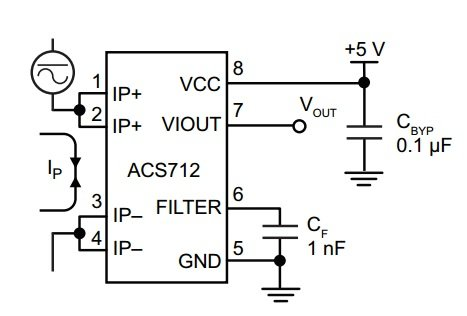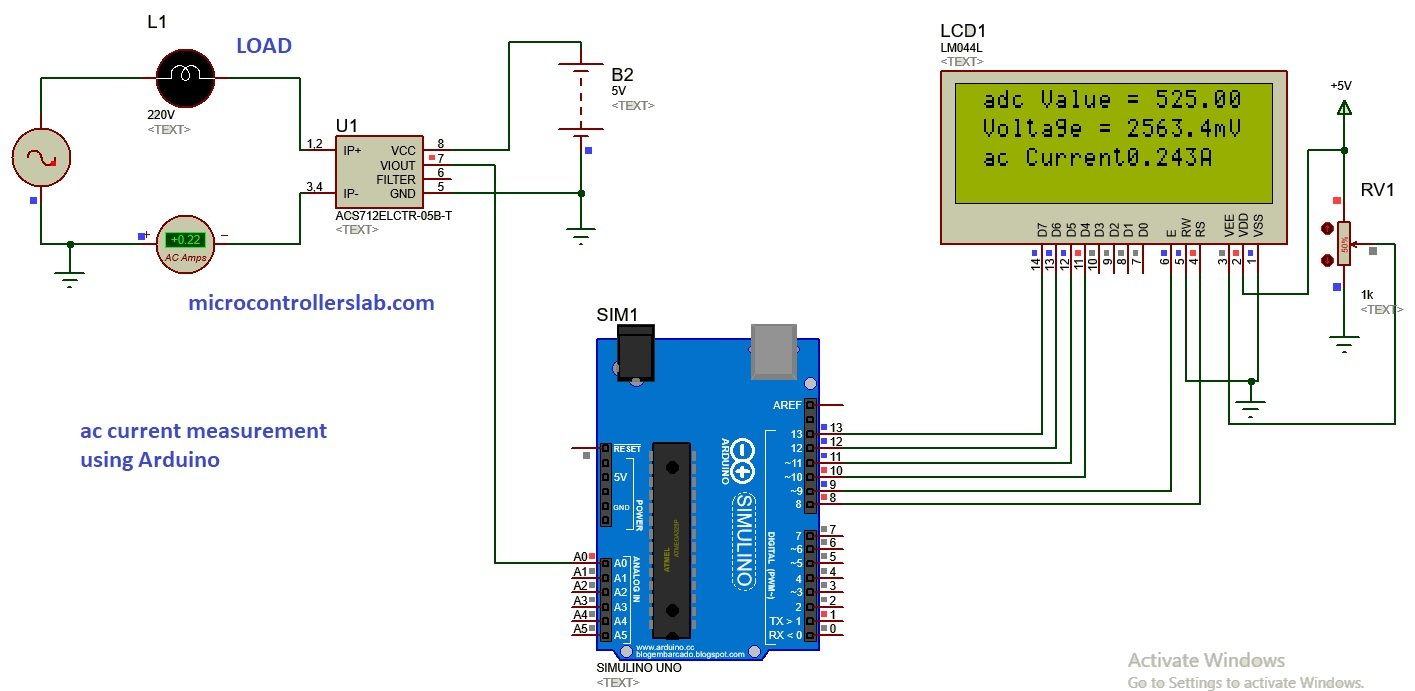# ac current measurement using acs712 hall effect current sensor and Arduino

Ac current measurement using acs712 hall effect current sensor and Arduino. In this tutorial, you will learn how to design alternating current measurement system using acs712 hall effect current sensor and Arduino Uno R3. But you can apply same concept to Arduino mega, pic microcontroller, 8051 microcontroller or avr microcontroller. you just need make change in code but the rest of the concept will remain same. In my last tutorial, I have shown how to measure dc current using acs712 current sensor. In that tutorial, I have also explained introduction to acs712 hall effect current sensor, working of current sensor and how to measure current from hall effect sensor.  I will apply same concepts in this article to measure ac current with acs712 hall effect current sensor.  you may also like to read how to measure ac voltage using Arduino.

## How to measure ac current with acs712 hall effect current sensor

As I have discussed in my last article, acs712 current sensor converts input current into proportional voltage depending on nature of the input current. If input current is dc, the output voltage of current sensor will be dc also. if input current to current sensor is ac, the output voltage of current sensor will be also alternating voltage. Shape of output voltage depend on the shape of input current. if shape of input current is sine wave, then the shape of output voltage will be also sine wave which is usually happens in case of ac current.  The pin out of hall effect current sensor is given below.so we will get sine wave at the output of hall effect sensor. So we need a method to measure peak of sine wave and then we can convert this peak voltage back into rms voltage by simply multiplying it with square root of 2 which is the relationship between peak value and rms value of voltage. I have explained it more details in my last article on interfacing of acs712 hall effect current sensor with Arduino. I recommend you to read it first, if you want to understand, how to measure this output voltage and how to convert this output voltage back into current using Arduino.

## Ac current measurement using acs712 current sensor and Arduino

Circuit diagram for ac current measurement using acs712 current and Arduino is given below:## Code for ac current measurement using acs712 current sensor

``````// include the library code:
#include <LiquidCrystal.h> //library for LCD

// initialize the library with the numbers of the interface pins
LiquidCrystal lcd(8, 9, 10, 11, 12, 13);

//Measuring Current Using ACS712

const int analogchannel = 0; //Connect current sensor with A0 of Arduino
int sensitivity = 185; // use 100 for 20A Module and 66 for 30A Module
int offsetvoltage = 2500;
double Voltage = 0; //voltage measuring
double ecurrent = 0;// Current measuring

void setup() {
//baud rate
Serial.begin(9600);//baud rate at which arduino communicates with Laptop/PC
// set up the LCD's number of columns and rows:
lcd.begin(20, 4); //LCD order
// Print a message to the LCD.
lcd.setCursor(1,1);//Setting cursor on LCD
lcd.print("MICROCONTROLLERSLAB");//Prints on the LCD
lcd.setCursor(4,2);
lcd.print(".com");
delay(3000);//time delay for 3 sec
lcd.clear();//clearing the LCD display
lcd.display();//Turning on the display again
lcd.setCursor(1,0);//setting LCD cursor
lcd.setCursor(1,1);
lcd.print("DC Current Sensor");
lcd.setCursor(5,2);
lcd.print("ACS 712");
delay(2000);//delay for 2 sec
}

void loop() //method to run the source code repeatedly
{
unsigned int temp=0;
float maxpoint = 0;
int i=0;
for(i=0;i<500;i++)
{
{
maxpoint = temp;
}
}
Voltage = (adcvalue / 1024.0) * 5000; // Gets you mV
ecurrent = ((Voltage - offsetvoltage) / sensitivity);
ecurrent = ( ecurrent ) / ( sqrt(2) );
//Prints on the serial port
Serial.print("Raw Value = " ); // prints on the serial monitor
Serial.print(adcvalue); //prints the results on the serial monitor

lcd.clear();//clears the display of LCD
delay(1000);//delay of 1 sec
lcd.display();
lcd.setCursor(1,0);
lcd.setCursor(13,0);

Serial.print("\t mV = "); // shows the voltage measured
Serial.print(Voltage,3); // the '3' after voltage allows you to display 3 digits after decimal point

lcd.setCursor(1,1);
lcd.print("Voltage = ");
lcd.setCursor(11,1);
lcd.print(Voltage,3);
lcd.setCursor(17,1);
lcd.print("mV");//Unit for the voltages to be measured

Serial.print("\t ecurrent = "); // shows the voltage measured
Serial.println(ecurrent,3);// the '3' after voltage allows you to display 3 digits after decimal point

lcd.setCursor(1,2);
lcd.print("ac Current = ");
lcd.setCursor(11,2);
lcd.print(ecurrent,3);
lcd.setCursor(16,2);
lcd.print("A"); //unit for the current to be measured
delay(2500); //delay of 2.5 sec
}``````

In above code, I have taken 500 samples of output voltage of current sensor and detect the peak value of sine wave. After detecting peak value of voltage, it is converted back into current using the sensitivity formula given in code.

### 20 thoughts on “ac current measurement using acs712 hall effect current sensor and Arduino”

1. How come the value of my circuit keep increasing?

2. How can we measure the ac Voltage using ACS712 sensor?

3. Hello,
Great Explanation & Job Done.
But what is that 5000 in the line
” Voltage = (adcvalue / 1024.0) * 5000; // Gets you mV ”
Ravi

4. hi

can me tell me the code and simulation for converting analog speed of stepper motor into digital frequency pulses using ardunio

nandhini

5. i have a problem in simulating proteus .as i write a code for voltage measurement and sketch the desired circuitry in proteus but the A0 pin doesn’t read the value .Kindly if u have any idea ,i will be very thankful

• I think there is a bug in the libraries which you have downloaded from the website delete those library files and again re download them so that you can get the output

6. while running this coding in proteus im getting this error message.. how can i solve this ??

“Invalid opcode 0x9419 at PC=0x1890 “

7. does this coding valid for dc current ?

8. Sir, is this circuit measures the current in zero when we turn off ac power supply?
If no
Then. Why?

If yes

Then How.

9. where is the code?

10. i have simulated this program. but value in screen does not match with original value & also if i change the input current no change in display

• You need to make sure, you have used a correct connection with ADC channel of Arduino

11. Sir..I want to show 220v and 50mhz.Whats the formula..?thank’s.

12. This is buggy code. It ignores Nyquist Sampling theorem. Simply measuring 500 times without any knowledge of what is measured gives an result you cannot trust. The 500 count loop is executed in few ms. The period of AC (50Hz) is 20 ms. So you’re sampling your AC for a fraction of the period of the AC. The maxvalue in those 500 points can be any value. And if just ‘any’ value is measured, nothing is measured. You need an algoritm to measure te RMS-value. You need to measure for more than 20 ms at a sample rate higher than 100 Hz. Above that it is advised to use the same reference voltage for the ADC in the AVR and the ACS.

• For your kind information, we are getting each sample every 104 µs which is a default sampling time of the analogRead() function of Arduino. By taking 500 samples we make sure that we do not miss a peak value and check it for 2-3 AC cycles. For your second concern, 500*0.104ms = 50ms which not a fraction of the AC period. You should have done the homework first before commenting on this post.

13. Hi, I have tried to simulate the circuit using the above code. However, the reading on the AC AmMeter and the value display on the LCD do not match each other. Check back both the circuit and the code but nothing seems to be out of order. Is there any recommendation?

Thank you very much.

• This is the code I have been implementing. Of course, I tried to apply your method into my simulation using Proteus 8.8 but I seem to not the desired results..

// include the library code:
#include //library for LCD

// initialize the library with the numbers of the interface pins
LiquidCrystal lcd(8, 9, 10, 11, 12, 13);

//Measuring Current Using ACS712

const int analogchannel = A0; //Connect current sensor with A0 of Arduino
int sensitivity = 185; // use 100 for 20A Module and 66 for 30A Module
int offsetvoltage = 2500;
double Voltage = 0; //voltage measuring
double ecurrent = 0; //current measuring
double power = 0; //power measuring

void setup() {
//baud rate
Serial.begin(9600);//baud rate at which arduino communicates with Laptop/PC
// set up the LCD’s number of columns and rows:
lcd.begin(20, 4); //LCD order
}

void loop() //method to run the source code repeatedly
{
unsigned int temp = 0;
float maxpoint = 0;
int i = 0;
for(i = 0; i maxpoint)
{
maxpoint = temp;
}
}
Voltage = (adcvalue / 1024.0) * 5000; // Gets you mV
ecurrent = ((Voltage – offsetvoltage) / sensitivity);
ecurrent = ( ecurrent ) / ( sqrt(2) );

power = (ecurrent*220/1.13);

//Prints on the serial port
Serial.print(“Raw Value = ” ); // prints on the serial monitor
Serial.print(adcvalue); // prints the results on the serial monitor

lcd.clear(); // clears the display of LCD
delay(1000); // delay of 1 sec
lcd.display();
lcd.setCursor(1,0);
lcd.setCursor(13,0);

Serial.print(“\t mV = “); // shows the voltage measured
Serial.print(Voltage,3); // the ‘3’ after voltage allows you to display 3 digits after decimal point

lcd.setCursor(1,1);
lcd.print(“Voltage = “);
lcd.setCursor(11,1);
lcd.print(Voltage,3);
lcd.setCursor(18,1);
lcd.print(“mV”); //Unit for the voltages to be measured

Serial.print(“\t ecurrent = “); // shows the current measured
Serial.println(ecurrent,3); // the ‘3’ after current allows you to display 3 digits after decimal point

lcd.setCursor(1,2);
lcd.print(“Current = “);
lcd.setCursor(11,2);
lcd.print(ecurrent,3);
lcd.setCursor(16,2);
lcd.print(“A”); //unit for the current to be measured

Serial.print(“\t power = “); // shows the power measured
Serial.println(power,3); // the ‘3’ after power allows you to display 3 digits after decimal point

lcd.setCursor(1,3);
lcd.print(“Power = “);
lcd.setCursor(10,3);
lcd.print(power,3);
lcd.setCursor(18,3);
lcd.print(“W”); //unit for the power to be measured

delay(2500); //delay of 2.5 sec
}